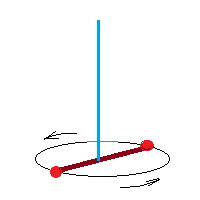## Friday, May 7, 2021

### Rotational Oscillation: UNIZOR.COM - Physics4Teens - Waves - Mechanical ...

Notes to a video lecture on http://www.unizor.com

Rotational Oscillation

Rotational oscillations (also called torsional oscillations) can be observed in movements of a balance wheel inside hand watches. It rotates, that's why it's rotational, and it moves along the same trajectory back and forth, that's why it's oscillation.

Another example might be a weightless horizontal rod with two identical weights at its opposite ends hanging on a vertical steel wire attached to a rod's midpoint.If we wind up the horizontal rod, as shown on the picture, and let it go, it will create a tension in the twisted wire that will start untwisting, returning the rod into its original position, then winding in an opposite direction etc., thus oscillating rotationally.

Recall the concept of a torque for rotational movement
τ = R·F
In a simple case of a force acting perpendicularly to a radius (the only case we will consider) the above can be interpreted just as a multiplication. In a more general case, assuming both force and radius are vectors, the above represents a vector product of these vectors, making a torque also a vector.

While the tension force of a twisted steel wire F1 might be significant, it acts on a very small radius of a wire r, so the force F2, acting on each of two weights on opposite sides of a rod of radius R and having the same torque τ, is proportionally weaker
τ = r·F1 = R·F2
from which follows
F1 /F2 = R /r
and
F2 = (r/R)·F1 = τ /R

Dynamics of reciprocating (back and forth) movement are expressed in terms of inertial mass m, force F and acceleration a by the Second Newton's Law
F = m·a

In case of a rotational movement with a radius of rotation R the dynamics are expressed in terms of moment of inertia I=m·R², torque τ=R·F and angular acceleration α=a/R by the rotational equivalent of the Second Newton's Law
τ = I·α

There is a rotational equivalent of a Hooke's Law. It relates a torque τ and an angular displacement φ from a neutral (untwisted) position
τ = −k·φ

For rotational oscillations an angular displacement φ is a function of time φ(t). Angular acceleration α is a second derivative of an angular displacement φ(t).

Therefore, we can equate the torque expressed according to the rotational equivalent of the Second Newton's Law to the one expressed according to the rotational equivalent of the Hooke's law, getting an equation
I·α = −k·φ
or
I·φ"(t) = −k·φ(t)
or
φ"(t) = −(k/I)·φ(t)

The differential equation above is of the same type as for an oscillations of a weight on a spring discussed in the previous lecture. The only difference is that, instead of a mass of an object m we use moment of inertia I=m·R².

For initial angular displacement (initial twist) of a steel wire φ(0)=γ and no initial angular speed (φ'(0)=0) the solution to this equation is
φ(t) = γ·cos(√k/I·t)

The rotational oscillations in our case have a period (the shortest time the object returns to its original position)
T = 2π·√I/k

Frequency of rotational oscillations f = 1/T.
Therefore, our rod with two weights makes
f = 1/T = (1/2π)·√k/I
oscillations per second.

Since I=m·R², the period is greater (and the frequency is smaller) when objects are more massive and on a greater distance from a center of a rod, where the wire is attached.

Notice that a period and a frequency of these oscillations are not dependent on initial angle of turning the rod φ(0)=γ. This parameter γ defines only the amplitude of oscillations, but not their period and frequency.

This is an important factor used, for example, in watch making with a balance wheel oscillating based on its physical characteristics and an elasticity of a spiral spring.
No matter how hard you wind a spring (or how weak it becomes after it worked for awhile), a balance wheel will maintain the same period and frequency of its oscillations.